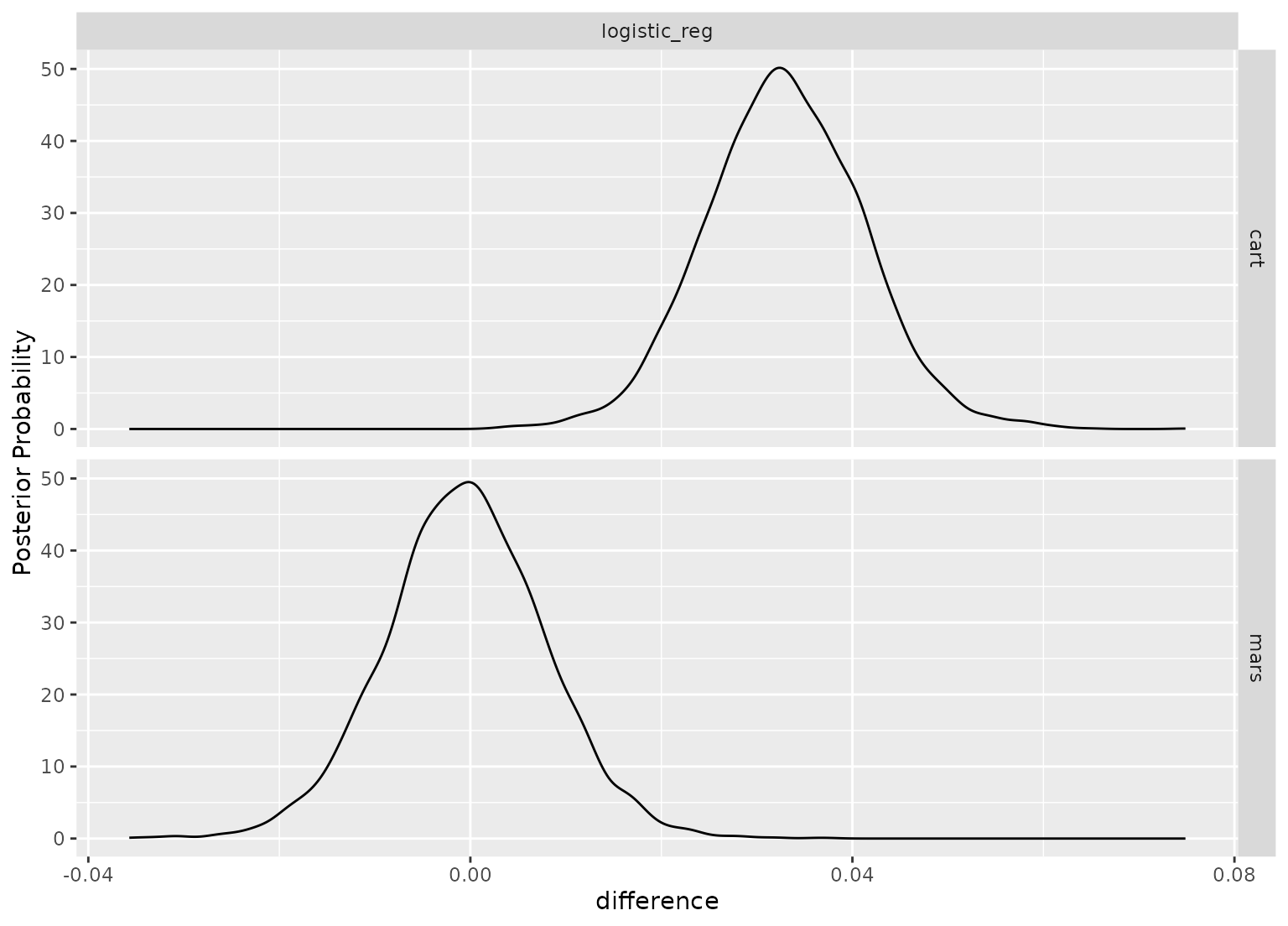A density is created for each contrast in a faceted grid.

# S3 method for posterior_diff
autoplot(object, size = 0, ...)

## Arguments

object An object produced by contrast_models(). The size of an effective difference. For example, a 5\ "real" difference. Options passed to geom_line(stat = "density", ...).

## Value

A ggplot2::ggplot() object using geom_density faceted by the models being contrasted (when there are 2 or more contrasts).

## Examples

data(ex_objects)
library(ggplot2)
autoplot(contrast_samples)